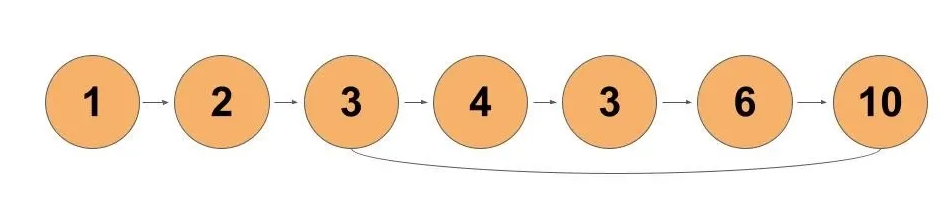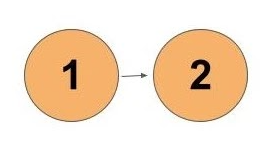# Starting point of loop in a Linked List

In this article, we will learn how to solve the most asked coding interview question: “Starting point of the loop in a Linked List

Problem Statement: Given the head of a linked list, return the node where the cycle begins. If there is no cycle, return null.

There is a cycle in a linked list if there is some node in the list that can be reached again by continuously following the next pointer. Internally, pos is used to denote the index of the node that the tail’s next pointer is connected to (0-indexed). It is -1 if there is no cycle. Note that pos is not passed as a parameter.

Do not modify the linked list.

Examples:

```Example 1:
Input:
Output:
tail connects to node index 2
Explanation:```
```Example 2:
Input:
Output:
no cycle
Explanation:```

Solution:

Solution 1: Brute Force

Approach:

We can store nodes in a hash table so that, if a loop exists, the head will encounter the same node again. This node will be present in the table and hence, we can detect the loop. The steps are:-

• Iterate the given list.
• For each node visited by the head pointer, check if the node is present in the hash table.
• If yes, the loop detected
• If not, insert the node in the hash table and move the head pointer ahead.
• If the head reaches null, then the given list does not have a cycle in it.

Dry Run:

We start iterating each node and storing nodes in the hash table if an element is not present.

Node(1) is not present in the hash table. So, we insert a node in it and move head ahead.

Node(2) is not present in the hash table. So, we insert a node in it and move head ahead.

Node(3) is not present in the hash table. So, we insert a node in it and move head ahead.

Node(4) is not present in the hash table. So, we insert a node in it and move head ahead.

Node(3) is not present in the hash table. So, we insert a node in it and move head ahead. Though this node contains 3 as a value, it is a different node than the node at position 3.

Node(6) is not present in the hash table. So, we insert a node in it and move head ahead.

Node(10) is not present in the hash table. So, we insert a node in it and move head ahead.

We reached the same node which was present in the hash table. Thus, the starting node of the cycle is node(3).

Code:

## C++ Code

``````#include<bits/stdc++.h>
using namespace std;

class node {
public:
int num;
node* next;
node(int val) {
num = val;
next = NULL;
}
};

node* newNode = new node(val);
return;
}
while(temp->next != NULL) temp = temp->next;

temp->next = newNode;
return;
}

int cnt = 0;
while(temp->next != NULL) {
if(cnt != pos) {
++cnt;
ptr = ptr->next;
}
temp = temp->next;
}
temp->next = ptr;
}
//process as per mentioned in solution
unordered_set<node*> st;
}
return NULL;
}

int main() {

if(nodeRecieve == NULL) cout<<"No cycle";
else {
int pos = 0;
while(temp!=nodeRecieve) {
++pos;
temp = temp->next;
}
cout<<"Tail connects at pos "<<pos<<endl;
}

return 0;
}
``````

Output: Tail connects at pos 2

Time Complexity: O(N)

Reason: Iterating the entire list once.

Space Complexity: O(N)

Reason: We store all nodes in a hash table.

## Java Code

``````import java.util.*;
class Node {
int num;
Node next;
Node(int val) {
num = val;
next = null;
}
}
class TUF{
static Node insertNode(Node head,int val) {
Node newNode = new Node(val);
}
while(temp.next != null) temp = temp.next;

temp.next = newNode;
}

static void createCycle(Node head,int pos) {
int cnt = 0;
while(temp.next != null) {
if(cnt != pos) {
++cnt;
ptr = ptr.next;
}
temp = temp.next;
}
temp.next = ptr;
}
//process as per mentioned in solution
HashSet<Node> st=new HashSet<>();
}
return null;
}

public static void main(String args[]) {

if(nodeRecieve == null) System.out.println("No cycle");
else {
int pos = 0;
while(temp!=nodeRecieve) {
++pos;
temp = temp.next;
}
System.out.println("Tail connects at pos "+pos);
}

}
}
``````

Output: Tail connects at pos 2

Time Complexity: O(N)

Reason: Iterating the entire list once.

Space Complexity: O(N)

Reason: We store all nodes in a hash table.

## Python Code

``````class Node:
def __init__(self, val):
self.val = val
self.next = None

# utility function to insert node at the end of the linked list
newNode = Node(val)
while temp.next != None:
temp = temp.next
temp.next = newNode

# utility function to create cycle
cnt = 0
while temp.next != None:
if cnt != pos:
ptr = ptr.next
cnt += 1
temp = temp.next
temp.next = ptr

# process as per mentioned in solution
st = set()
return None

if __name__ == "__main__":
if nodeRecieve == None:
print("No cycle")
else:
pos = 0
while temp != nodeRecieve:
pos += 1
temp = temp.next
print("Tail connects at pos", pos)``````

Output: Tail connects at pos 2

Time Complexity: O(N)

Reason: Iterating the entire list once.

Space Complexity: O(N)

Reason: We store all nodes in a hash table.

Solution 2: Slow and Fast Pointer Method

Approach:

The following steps are required:

• Initially take two pointers, fast and slow. The fast pointer takes two steps ahead while the slow pointer will take a single step ahead for each iteration.
• We know that if a cycle exists, fast and slow pointers will collide.
• If the cycle does not exist, the fast pointer will move to NULL
• Else, when both slow and fast pointer collides, it detects a cycle exists.
• Take another pointer, say entry. Point to the very first of the linked list.
• Move the slow and the entry pointer ahead by single steps until they collide.
• Once they collide we get the starting node of the linked list.

But why use another pointer, or xentry?

Let’s say a slow pointer covers the L2 distance from the starting node of the cycle until it collides with a fast pointer. L1 is the distance traveled by the entry pointer to the starting node of the cycle. So, in total, the slow pointer covers the L1+L2 distance. We know that a fast pointer covers some steps more than a slow pointer. Therefore, we can say that a fast pointer will surely cover the L1+L2 distance. Plus, a fast pointer will cover more steps which will accumulate to nC length where cC is the length of the cycle and n is the number of turns. Thus, the fast pointer covers the total length of L1+L2+nC.

We know that the slow pointer travels twice the fast pointer. So this makes the equation to

2(L1+L2) = L1+L2+nC. This makes the equation to

L1+L2 = nC. Moving L2 to the right side

L1 = nC-L2 and this shows why the entry pointer and the slow pointer would collide.

Dry Run:

We initialize fast and slow pointers to the head of the list. Fast moves two steps ahead and slowly takes a single step ahead.

We can see that the fast and slow pointer collides which shows the cycle exists. The entry pointer is pointed to the head of the list. And move them forward until it collides with the slow pointer.

We see that both collide and hence, we get the starting node of the list.

Code:

## C++ Code

``````#include<bits/stdc++.h>
using namespace std;

class node {
public:
int num;
node* next;
node(int val) {
num = val;
next = NULL;
}
};

node* newNode = new node(val);
return;
}
while(temp->next != NULL) temp = temp->next;

temp->next = newNode;
return;
}

int cnt = 0;
while(temp->next != NULL) {
if(cnt != pos) {
++cnt;
ptr = ptr->next;
}
temp = temp->next;
}
temp->next = ptr;
}
//process as per mentioned in solution

while(fast->next != NULL&&fast->next->next != NULL) {
slow = slow->next;
fast = fast->next->next;

if(slow == fast) {
while(slow != entry) {
slow = slow->next;
entry = entry->next;
}
return slow;
}
}
return NULL;
}

int main() {

if(nodeRecieve == NULL) cout<<"No cycle";
else {
int pos = 0;
while(temp!=nodeRecieve) {
++pos;
temp = temp->next;
}
cout<<"Tail connects at pos "<<pos<<endl;
}

return 0;
}
``````

Output: Tail connects at pos 2

Time Complexity: O(N)

Reason: We can take overall iterations and club them to O(N)

Space Complexity: O(1)

Reason: No extra data structure is used.

## Java Code

``````import java.util.*;
class Node {
int num;
Node next;
Node(int val) {
num = val;
next = null;
}
}
class TUF{
static Node insertNode(Node head,int val) {
Node newNode = new Node(val);
}
while(temp.next != null) temp = temp.next;

temp.next = newNode;
}

static void createCycle(Node head,int pos) {
int cnt = 0;
while(temp.next != null) {
if(cnt != pos) {
++cnt;
ptr = ptr.next;
}
temp = temp.next;
}
temp.next = ptr;
}
//process as per mentioned in solution

while(fast.next != null&&fast.next.next != null) {
slow = slow.next;
fast = fast.next.next;

if(slow == fast) {
while(slow != entry) {
slow = slow.next;
entry = entry.next;
}
return slow;
}
}
return null;
}

public static void main(String args[]) {

if(nodeRecieve == null) System.out.println("No cycle");
else {
int pos = 0;
while(temp!=nodeRecieve) {
++pos;
temp = temp.next;
}
System.out.println("Tail connects at pos "+pos);
}

}
}
``````

Output: Tail connects at pos 2

Time Complexity: O(N)

Reason: We can take overall iterations and club them to O(N)

Space Complexity: O(1)

Reason: No extra data structure is used.

## Python Code

``````class Node:
def __init__(self, val):
self.val = val
self.next = None

# utility function to insert node at the end of the linked list
newNode = Node(val)
while temp.next != None:
temp = temp.next
temp.next = newNode

# utility function to create cycle
cnt = 0
while temp.next != None:
if cnt != pos:
ptr = ptr.next
cnt += 1
temp = temp.next
temp.next = ptr

# process as per mentioned in solution
return None
while fast.next != None and fast.next.next != None:
slow = slow.next
fast = fast.next.next
if slow == fast:
while slow != entry:
slow = slow.next
entry = entry.next
return slow
return None

if __name__ == "__main__":
if nodeRecieve == None:
print("No cycle")
else:
pos = 0
while temp != nodeRecieve:
pos += 1
temp = temp.next
print("Tail connects at pos", pos)``````

Output: Tail connects at pos 2

Time Complexity: O(N)

Reason: We can take over all iterations and club them to O(N)

Space Complexity: O(1)

Reason: No extra data structure is used.